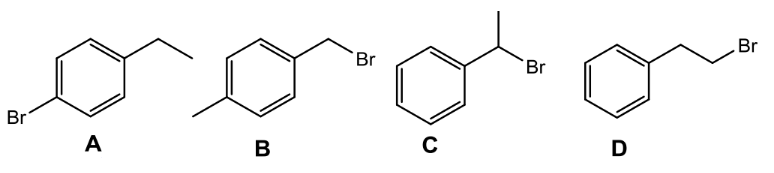# Problem: Which of the following structures with molecular formula C8H9Br is consistent with the the data below: 1H NMR: 1.3δ (triplet, I=3H), 2.65δ (quartet, I=2H), 7.2δ (doublet, I=2H), 7.5δ (doublet, I=2H)?

###### FREE Expert Solution
90% (225 ratings)
###### Problem Details

Which of the following structures with molecular formula C8H9Br is consistent with the the data below:

1H NMR: 1.3δ (triplet, I=3H), 2.65δ (quartet, I=2H), 7.2δ (doublet, I=2H), 7.5δ (doublet, I=2H)?What scientific concept do you need to know in order to solve this problem?

Our tutors have indicated that to solve this problem you will need to apply the Structure Determination without Mass Spect concept. You can view video lessons to learn Structure Determination without Mass Spect. Or if you need more Structure Determination without Mass Spect practice, you can also practice Structure Determination without Mass Spect practice problems.

What is the difficulty of this problem?

Our tutors rated the difficulty ofWhich of the following structures with molecular formula C8H...as medium difficulty.

How long does this problem take to solve?

Our expert Organic tutor, Nicolle took 3 minutes and 10 seconds to solve this problem. You can follow their steps in the video explanation above.

What professor is this problem relevant for?

Based on our data, we think this problem is relevant for Professor Daoudi's class at UCF.